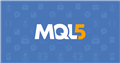# Custom indicator not plotting new Candle Pattern(s) once loaded on Chart

Dear Fellows

I am trying to create custom indicator iCandlePatterns.mq5 for identifying patterns. The Indicators works fine when loaded for the first time on chart and finds patterns for all the history data.

However, after that it does not update patterns as new candles are formed. I could not find what is wrong in my code, so here I am seeking assistance from Gurus.

```int OnCalculate(const int               rates_total,
const int               prev_calculated,
const datetime  	&time[],
const double            &open[],
const double            &high[],
const double            &low[],
const double            &close[],
const long              &tick_volume[],
const long              &volume[],

// Set price and other buffers AsSeries
ArraySetAsSeries(open,true);                                                            ArraySetAsSeries(high,true);
ArraySetAsSeries(low,true);                                                             ArraySetAsSeries(close,true);
ArraySetAsSeries(time,true);                                                            ArraySetAsSeries(tick_volume,true);

// Check if there are enough bars for calculation
if(rates_total < SkipBars)                                                              return(0);

// How many bars to calculate
int count = rates_total - SkipBars - prev_calculated;                                   // number of bars not yet calculated
if(prev_calculated > 0)         count++;                                                // force last calculated bar to be done again

// Main indicator calculation loop
for(int i = count-1; i >= 0; i--) {

b3BC[i]        = is3BlackCrows(open,high,low,close,tick_volume,time,i)          ? low[i]  : EMPTY_VALUE;
bBearDoji[i]   = EMPTY_VALUE;
bHammer[i]     = isHammer(open,high,low,close,tick_volume,time,i)               ? low[i]  : EMPTY_VALUE;
bPiercing[i]   = isPiercing(open,high,low,close,tick_volume,time,i)             ? low[i]  : EMPTY_VALUE;
bBullInside[i] = isBullInside(open,high,low,close,tick_volume,time,i)           ? low[i]  : EMPTY_VALUE;
bBullEngulf[i] = isBullEngulf(open,high,low,close,tick_volume,time,i)           ? low[i]  : EMPTY_VALUE;
bBullStrong[i] = EMPTY_VALUE;

b3WS[i]        = is3WhiteSoldiers(open,high,low,close,tick_volume,time,i) 	? high[i] : EMPTY_VALUE;
bBullDoji[i]   = EMPTY_VALUE;
bSStar[i]      = isSStar(open,high,low,close,tick_volume,time,i)                ? high[i] : EMPTY_VALUE;
bDCCover[i]    = isDCCover(open,high,low,close,tick_volume,time,i)              ? high[i] : EMPTY_VALUE;
bBearInside[i] = isBearInside(open,high,low,close,tick_volume,time,i)           ? high[i] : EMPTY_VALUE;
bBearEngulf[i] = isBearEngulf(open,high,low,close,tick_volume,time,i)           ? high[i] : EMPTY_VALUE;
bBearStrong[i] = EMPTY_VALUE;
}

// Return value for next bar
return(rates_total);

} // End of function OnCalculate()
```

I have also created a class CCandlePatterns.mqh to get data in EA or Strategies. The is3BC() and is3WS() methods are returning false values even when the pattern is found by indicator.

It seems as indicator values are not updated, is3BC() method get a 'Zero' value instead of EMPTY_VALUES, and hence get a false result. If any other problem is causing this, I would request to help me on that too.

Below is the result from EATest run:

```2023.11.14 16:36:11.869 2023.11.08 15:00:00   [US30,PERIOD_H1] CCPatterns::is3BC [2023.11.08 14:00] Index[0.00] [0.00]            ... This bar has 3BC Pattern
2023.11.14 16:36:11.869 2023.11.08 15:00:00   [US30,PERIOD_H1] CCPatterns::is3BC [2023.11.08 13:00] Index[0.00] [0.00]
2023.11.14 16:36:11.869 2023.11.08 15:00:00   [US30,PERIOD_H1] CCPatterns::is3BC [2023.11.08 12:00] Index[0.00] [0.00]
2023.11.14 16:36:11.869 2023.11.08 15:00:00   is3BC[false] [false] [false]

2023.11.14 16:36:16.400 2023.11.08 20:00:00   [US30,PERIOD_H1] CCPatterns::is3WS [2023.11.08 19:00] Index[0.00] [0.00]            ... This bar has 3WS Pattern
2023.11.14 16:36:16.400 2023.11.08 20:00:00   [US30,PERIOD_H1] CCPatterns::is3WS [2023.11.08 18:00] Index[0.00] [0.00]
2023.11.14 16:36:16.400 2023.11.08 20:00:00   [US30,PERIOD_H1] CCPatterns::is3WS [2023.11.08 17:00] Index[0.00] [0.00]
2023.11.14 16:36:16.400 2023.11.08 20:00:00   is3WS[false] [false] [false]
```

Necessary files are attached herewith including the screen shot of Tester Run and Live Chart data.

Also guide me how can I check pattern once on each new bar, instead of every tick in OnCalculate() method.

Support from the members to figure it out is highly appreciated. Thanks a lot in advance.Documentation on MQL5: Technical Indicators / iCustom
• www.mql5.com
iCustom - Technical Indicators - MQL5 Reference - Reference on algorithmic/automated trading language for MetaTrader 5
Files:
MQL_Live.png  441 kb
MQL_Tester.png  433 kb

would it benefit your script if you would remove ArraysSetAsSeries in OnCalculate and instead make an incremental loop for the main loop?

```                // Set price and other buffers AsSeries //(removed)
//ArraySetAsSeries(open,true);                                                                                                                                  ArraySetAsSeries(high,true);
//ArraySetAsSeries(low,true);                                                                                                                                           ArraySetAsSeries(close,true);
//ArraySetAsSeries(time,true);                                                                                                                                  ArraySetAsSeries(tick_volume,true);

// Check if there are enough bars for calculation
//if(rates_total < SkipBars)                                                                                                                                            return(0);

// How many bars to calculate
int count = rates_total - prev_calculated - SkipBars;                                   // number of bars not yet calculated
//if(prev_calculated > 0)               count++;        //(removed)

// Main indicator calculation loop
for(int i = count; i < rates_total; i++) {
//code
}```

I'm not a Guru or anything, just making a suggestion.

Conor Mcnamara #:

would it benefit your script if you would remove ArraysSetAsSeries in OnCalculate and instead make an incremental loop for the main loop?

I'm not a Guru or anything, just making a suggestion.